UIHistories Project: A History of the University of Illinois by Kalev Leetaru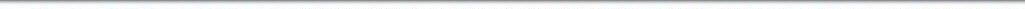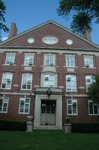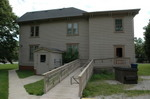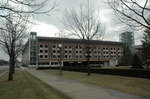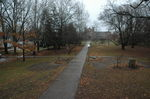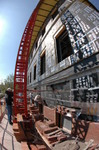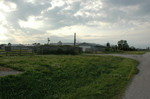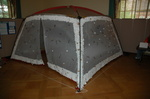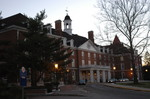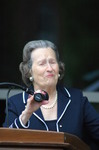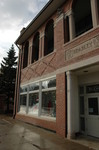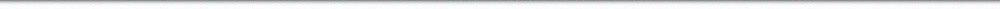N A V I G A T I O N D I G I T A L L I B R A R Y

## Repository: UIHistories Project: Board of Trustees Minutes - 1896 [PAGE 235]

Caption: Board of Trustees Minutes - 1896
This is a reduced-resolution page image for fast online browsing.

< Previous Page [Displaying Page 235 of 371] Next Page >
[VIEW ALL PAGE THUMBNAILS]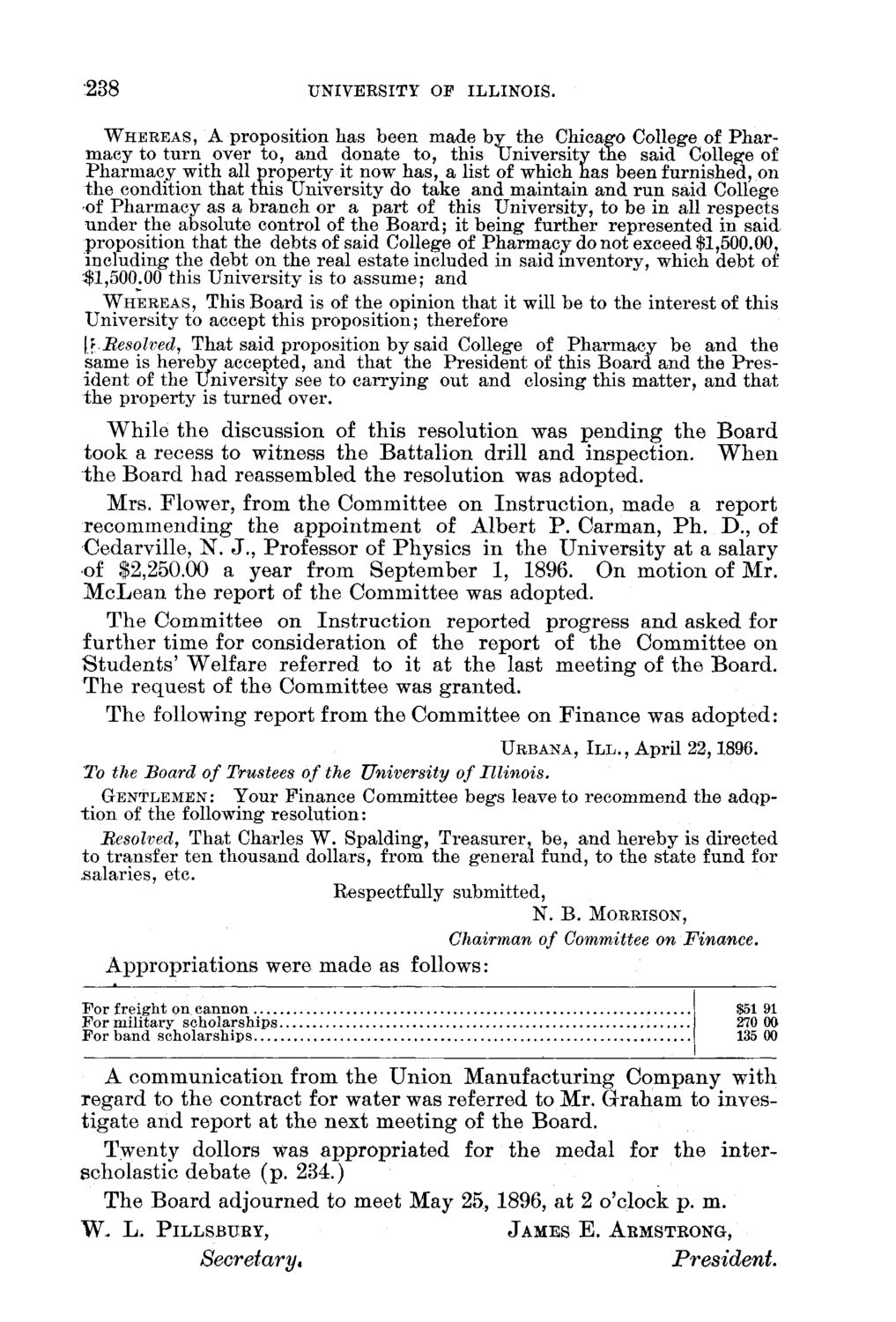### EXTRACTED TEXT FROM PAGE:

238

UNIVERSITY OF ILLINOIS.

WHEREAS, A proposition has been made by the Chicago College of Pharmacy to turn over to, and donate to, this University the said College of Pharmacy with all property it now has, a list of which has been furnished, on the condition that this University do take and maintain and run said College of Pharmacy as a branch or a part of this University, to be in all respects under the absolute control of the Board; it being further represented in said proposition that the debts of said College of Pharmacy do not exceed \$1,500.00, including the debt on the real estate included in said inventory, which debt of \$1,500.00 this University is to assume; and WHEREAS, This Board is of the opinion that it will be to the interest of this University to accept this proposition; therefore [r.Besqlved, That said proposition by said College of Pharmacy be and the same is hereby accepted, and that the President of this Board and the President of the university see to carrying out and closing this matter, and that the property is turned over. W h i l e t h e discussion of t h i s resolution was p e n d i n g t h e B o a r d took a recess t o witness t h e B a t t a l i o n drill a n d inspection. W h e n t h e B o a r d h a d reassembled trie r e s o l u t i o n was adopted. M r s . Flower, from t h e C o m m i t t e e o n I n s t r u c t i o n , m a d e a r e p o r t r e c o m m e n d i n g t h e a p p o i n t m e n t of A l b e r t P . C a r m a n , P h . D . , of Cedarville, N . J . , Professor of P h y s i c s i n t h e U n i v e r s i t y a t a salary of \$2,250.00 a year from S e p t e m b e r 1, 1896. O n m o t i o n of M r . M c L e a n t h e r e p o r t of t h e C o m m i t t e e was adopted. T h e C o m m i t t e e o n I n s t r u c t i o n r e p o r t e d progress a n d a s k e d for f u r t h e r t i m e for consideration of t h e r e p o r t of t h e C o m m i t t e e o n S t u d e n t s ' W e l f a r e referred t o i t a t t h e last m e e t i n g of t h e B o a r d . T h e r e q u e s t of t h e C o m m i t t e e was g r a n t e d . T h e following r e p o r t from t h e C o m m i t t e e on F i n a n c e was a d o p t e d :

URBANA, I I I . , April 22,1896.

To the Board of Trustees of the University of Illinois. GENTLEMEN: Your Finance Committee begs leave to recommend the adoption of the following resolution: Besolved, That Charles W. Spalding, Treasurer, be, and hereby is directed to transfer ten thousand dollars, from the general fund, to the state fund for salaries, etc. Respectfully submitted,

N. B . MORRISON,

Chairman of Committee on Finance. A p p r o p r i a t i o n s were m a d e as follows:

For freight on cannon For military scholarships For band scholarships

\$51 91 270 00 135 00

A c o m m u n i c a t i o n from t h e U n i o n M a n u f a c t u r i n g C o m p a n y w i t h r e g a r d t o t h e c o n t r a c t for water was referred to Mr. G r a h a m t o invest i g a t e a n d r e p o r t at t h e n e x t m e e t i n g of t h e B o a r d . T w e n t y dollors w a s a p p r o p r i a t e d for t h e m e d a l for t h e i n t e r scholastic d e b a t e ( p . 234.) T h e B o a r d adjourned t o meet M a y 25, 1896, a t 2 o'clock p . m.

W . L. PILLSBURY, JAMES E. ARMSTRONG,

Secretary*

President.